Putting a decimal point in a number causes arithmetic operations involving that number and rational numbers to be carried out in floating point. For example:

> 3.4 - 12/5;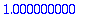An exception to this is that 0.0 is always simplified to the integer 0 . Thus you don't get a floating-point result by adding 0.0 to a rational.

> 3.4 - 17/5;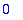> 0.0 + 17/5;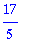Multiplying by 1.0 , on the other hand, should work, because 1.0 is not simplified to the integer 1 .

> 1.0 * 17/5;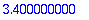However, even if the result is returned in floating point, it does not necessarily mean that the calculations were all done in floating point. In the following example, the calculation of (1+1/4321)^4321 is done in exact rational arithmetic, and then the result converted to floating point. You can tell that this is the case because (except on a very fast computer) the calculation takes a noticeable amount of time.

> 0.1*(1+1/4321)^4321;On the other hand, in the following case all calculations are done in floating point. The result is returned much more quickly (but is not as accurate due to roundoff error).

> (1+1.0/4321)^4321;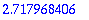Thus the statement "Putting a decimal point in a number causes arithmetic operations involving that number and rational numbers to be carried out in floating point" must be interpreted correctly. In evaluating an expression, Maple does a sequence of arithmetic operations (additions, multiplications, etc.). Those that directly involve a floating-point number (which may be a number written with a decimal point, or the result of another floating-point operation) are done in floating point, while those that only involve rational numbers are done using exact rational arithmetic. Matters may be complicated by the fact that the way Maple organizes these operations may be somewhat different from the way you write the expression. For example:

> p:= .333333: r:= 1000+p; r - 3001/3;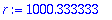> (1000+p)-3001/3;In the first calculation, Maple calculates r as a floating-point number. The fraction 3001/3 , when converted to floating point, becomes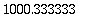as well, so the result is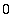. You might expect the same thing to happen in the second calculation, but it doesn't: it seems that Maple's preliminary simplification of the input ignores the parentheses and combines thewith the -3001/3 , so that the result is the same as

> p - 1/3;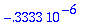The other way to cause a calculation to be done in floating point is to use evalf . However, this is also affected by automatic simplification, which may cause parts of the calculation to be done in exact rational arithmetic.

See also: Automatic simplification and evalf , Digits , evalf , Exact vs. floating-point computations , Order of operations in floating-point computation , Roundoff error , The meaning of Digits

Maple Advisor Database, R. Israel 1997